# 训练变分自编码器 (VAE) 以生成图像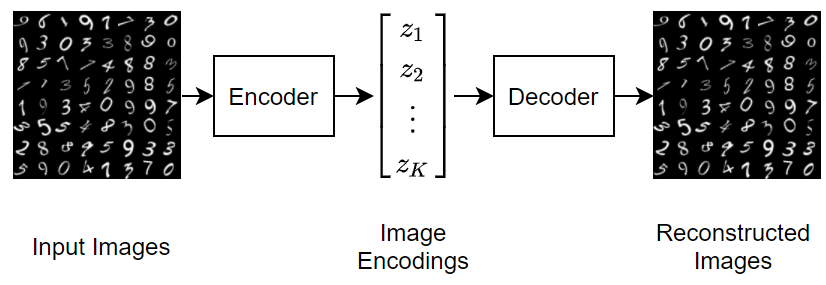### 加载数据

http://yann.lecun.com/exdb/mnist/ 下载训练和测试 MNIST 文件，并使用此示例附带的 `processImagesMNIST` 函数提取图像作为支持文件。要访问此函数，请以实时脚本形式打开此示例。VAE 不需要标注数据。

```trainImagesFile = "train-images-idx3-ubyte.gz"; testImagesFile = "t10k-images-idx3-ubyte.gz"; XTrain = processImagesMNIST(trainImagesFile);```
```Read MNIST image data... Number of images in the dataset: 60000 ... ```
`XTest = processImagesMNIST(testImagesFile);`
```Read MNIST image data... Number of images in the dataset: 10000 ... ```

### 定义网络架构

#### 定义编码器网络架构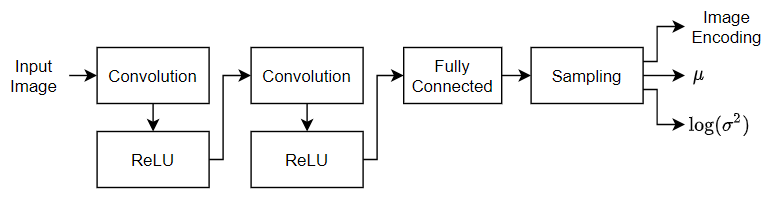• 对于图像输入，请指定输入大小与训练数据匹配的图像输入层。不要对数据进行归一化。

• 要对输入进行下采样，请指定对应于二维卷积层和 ReLU 层的两个块。

• 要输出均值和对数方差的串联向量，请指定一个输出通道数是潜在通道数的两倍的全连接层。

• 要对统计量指定的编码进行采样，请使用自定义层 `samplingLayer` 来包含一个采样层。要访问此层，请以实时脚本形式打开此示例。

```numLatentChannels = 16; imageSize = [28 28 1]; layersE = [ imageInputLayer(imageSize,Normalization="none") convolution2dLayer(3,32,Padding="same",Stride=2) reluLayer convolution2dLayer(3,64,Padding="same",Stride=2) reluLayer fullyConnectedLayer(2*numLatentChannels) samplingLayer];```

#### 定义解码器网络架构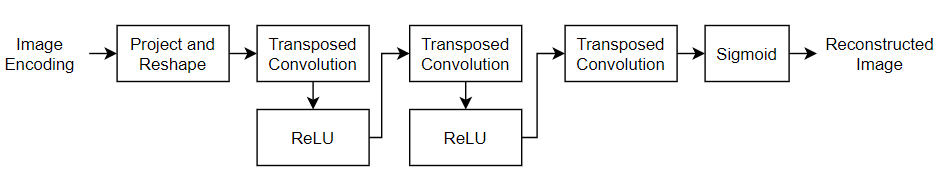• 对于特征向量输入，指定一个输入大小与潜在通道数匹配的特征输入层。

• 使用自定义层 `projectAndReshapeLayer`（该自定义层以支持文件的形式包含在此示例中）将潜在输入投影并重构为 7×7×64 数组。要访问此层，请以实时脚本形式打开此示例。将投影大小指定为 `[7 7 64]`

• 要对输入进行上采样，请指定对应于转置卷积层和 ReLU 层的两个块。

• 要输出大小为 28×28×1 的图像，请包含一个具有 3×3 滤波器的转置卷积层。

• 要将输出映射到 [0,1] 范围内的值，请包括 sigmoid 激活层。

```projectionSize = [7 7 64]; numInputChannels = size(imageSize,1); layersD = [ featureInputLayer(numLatentChannels) projectAndReshapeLayer(projectionSize) transposedConv2dLayer(3,64,Cropping="same",Stride=2) reluLayer transposedConv2dLayer(3,32,Cropping="same",Stride=2) reluLayer transposedConv2dLayer(3,numInputChannels,Cropping="same") sigmoidLayer];```

```netE = dlnetwork(layersE); netD = dlnetwork(layersD);```

### 定义模型损失函数

`modelLoss` 函数（在示例的模型损失函数部分中定义）接受编码器和解码器网络以及小批量输入数据作为输入，并返回损失以及损失关于网络中可学习参数的梯度。为了计算损失，该函数使用 `ELBOloss` 函数（在示例的 ELBO 损失函数部分中定义），接受编码器输出的均值和对数方差作为输入，并使用它们来计算证据下界 (ELBO) 损失。

### 指定训练选项

```numEpochs = 30; miniBatchSize = 128; learnRate = 1e-3;```

### 训练模型

• 将训练数据转换为数组数据存储。指定对第四个维度进行迭代。

• 使用自定义小批量预处理函数 `preprocessMiniBatch`（在此示例末尾定义）将多个观测值串联成单个小批量。

• 用维度标签 `"SSCB"`（空间、空间、通道、批量）格式化图像数据。默认情况下，`minibatchqueue` 对象将数据转换为基础类型为 `single``dlarray` 对象。

• 在 GPU 上（如果有）进行训练。默认情况下，如果 GPU 可用，则 `minibatchqueue` 对象会将每个输出都转换为一个 `gpuArray`。使用 GPU 需要 Parallel Computing Toolbox™ 和支持的 GPU 设备。有关受支持设备的信息，请参阅GPU Computing Requirements (Parallel Computing Toolbox)

• 为确保所有小批量都具有相同的大小，请丢弃任何不完整小批量。

```dsTrain = arrayDatastore(XTrain,IterationDimension=4); numOutputs = 1; mbq = minibatchqueue(dsTrain,numOutputs, ... MiniBatchSize = miniBatchSize, ... MiniBatchFcn=@preprocessMiniBatch, ... MiniBatchFormat="SSCB", ... PartialMiniBatch="discard");```

```trailingAvgE = []; trailingAvgSqE = []; trailingAvgD = []; trailingAvgSqD = [];```

```numObservationsTrain = size(XTrain,4); numIterationsPerEpoch = ceil(numObservationsTrain / miniBatchSize); numIterations = numEpochs * numIterationsPerEpoch;```

```monitor = trainingProgressMonitor( ... Metrics="Loss", ... Info="Epoch", ... XLabel="Iteration");```

• 使用 `dlfeval``modelLoss` 函数计算模型损失和梯度。

• 使用 `adamupdate` 函数更新编码器和解码器网络参数。

• 显示训练进度。

```epoch = 0; iteration = 0; % Loop over epochs. while epoch < numEpochs && ~monitor.Stop epoch = epoch + 1; % Shuffle data. shuffle(mbq); % Loop over mini-batches. while hasdata(mbq) && ~monitor.Stop iteration = iteration + 1; % Read mini-batch of data. X = next(mbq); % Evaluate loss and gradients. [loss,gradientsE,gradientsD] = dlfeval(@modelLoss,netE,netD,X); % Update learnable parameters. [netE,trailingAvgE,trailingAvgSqE] = adamupdate(netE, ... gradientsE,trailingAvgE,trailingAvgSqE,iteration,learnRate); [netD, trailingAvgD, trailingAvgSqD] = adamupdate(netD, ... gradientsD,trailingAvgD,trailingAvgSqD,iteration,learnRate); % Update the training progress monitor. recordMetrics(monitor,iteration,Loss=loss); updateInfo(monitor,Epoch=epoch + " of " + numEpochs); monitor.Progress = 100*iteration/numIterations; end end```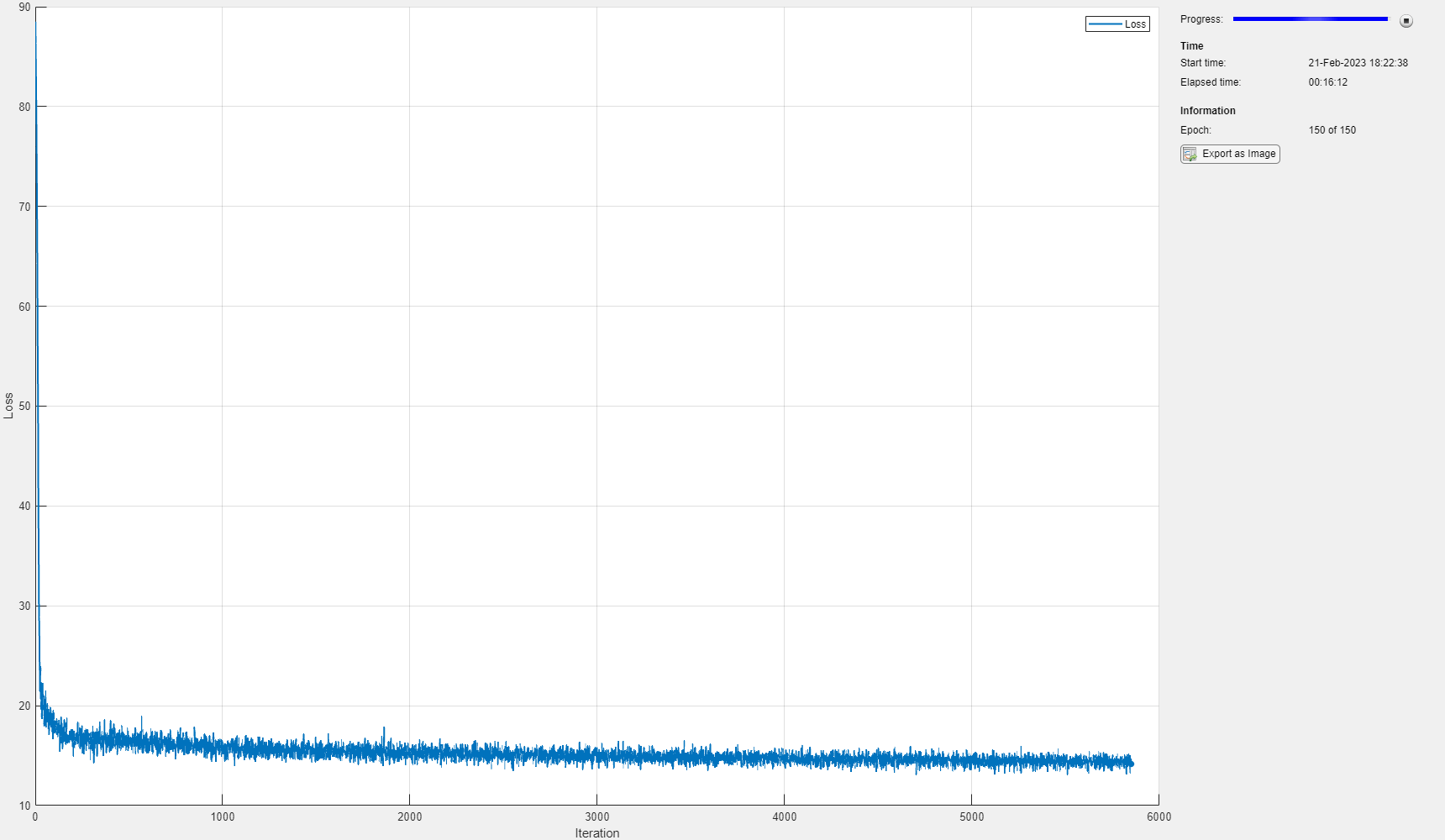### 测试网络

```dsTest = arrayDatastore(XTest,IterationDimension=4); numOutputs = 1; mbqTest = minibatchqueue(dsTest,numOutputs, ... MiniBatchSize = miniBatchSize, ... MiniBatchFcn=@preprocessMiniBatch, ... MiniBatchFormat="SSCB");```

`YTest = modelPredictions(netE,netD,mbqTest);`

```err = mean((XTest-YTest).^2,[1 2 3]); figure histogram(err) xlabel("Error") ylabel("Frequency") title("Test Data")```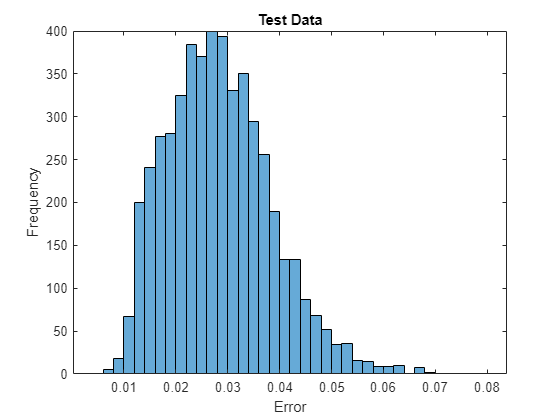### 生成新图像

```numImages = 64; ZNew = randn(numLatentChannels,numImages); ZNew = dlarray(ZNew,"CB"); YNew = predict(netD,ZNew); YNew = extractdata(YNew);```

```figure I = imtile(YNew); imshow(I) title("Generated Images")```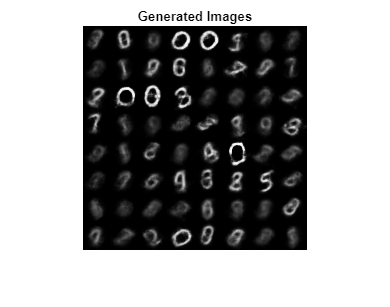### 辅助函数

#### 模型损失函数

`modelLoss` 函数接受编码器和解码器网络以及输入数据的一个小批量作为输入，并返回损失以及损失关于网络中可学习参数的梯度。该函数通过编码器传递训练图像，并通过解码器传递生成的图像编码。为了计算损失，该函数使用 `elboLoss` 函数以及编码器采样层输出的均值和对数方差统计量。

```function [loss,gradientsE,gradientsD] = modelLoss(netE,netD,X) % Forward through encoder. [Z,mu,logSigmaSq] = forward(netE,X); % Forward through decoder. Y = forward(netD,Z); % Calculate loss and gradients. loss = elboLoss(Y,X,mu,logSigmaSq); [gradientsE,gradientsD] = dlgradient(loss,netE.Learnables,netD.Learnables); end```

#### ELBO 损失函数

`ELBOloss` 函数接受编码器输出的均值和对数方差，并使用它们来计算证据下界 (ELBO) 损失。ELBO 损失由两个单独的损失项相加得出：

$\mathrm{ELBO}\text{}\mathrm{loss}=\mathrm{reconstruction}\text{}\mathrm{loss}+\mathrm{KL}\text{}\mathrm{loss}$.

$\mathrm{reconstruction}\text{}\mathrm{loss}=\mathrm{MSE}\left(\mathrm{reconstructed}\text{}\mathrm{image},\mathrm{input}\text{}\mathrm{image}\right)$.

KL 损失，即 Kullback–Leibler 散度，测量两个概率分布之间的差异。在本例中，最小化 KL 损失意味着确保学习的均值和方差尽可能接近目标（正态）分布的均值和方差。对于大小为 $\mathit{K}$ 的潜在维度，KL 损失的计算公式如下

$\mathrm{KL}\text{}\mathrm{loss}=-0.5\cdot \sum _{\mathit{i}=1}^{\mathit{K}}\left(1+\mathrm{log}\left({\sigma }_{\mathit{i}}^{2}\right)-{\mu }_{\mathit{i}}^{2}-{\sigma }_{\mathit{i}}^{2}\right)$.

```function loss = elboLoss(Y,T,mu,logSigmaSq) % Reconstruction loss. reconstructionLoss = mse(Y,T); % KL divergence. KL = -0.5 * sum(1 + logSigmaSq - mu.^2 - exp(logSigmaSq),1); KL = mean(KL); % Combined loss. loss = reconstructionLoss + KL; end```

#### 模型预测函数

`modelPredictions` 函数接受编码器和解码器网络对象以及输入数据 `mbq ``minibatchqueue` 作为输入，并通过迭代 `minibatchqueue` 对象中的所有数据来计算模型预测。

```function Y = modelPredictions(netE,netD,mbq) Y = []; % Loop over mini-batches. while hasdata(mbq) X = next(mbq); % Forward through encoder. Z = predict(netE,X); % Forward through dencoder. XGenerated = predict(netD,Z); % Extract and concatenate predictions. Y = cat(4,Y,extractdata(XGenerated)); end end```

#### 小批量预处理函数

`preprocessMiniBatch` 函数通过串联第四个维度上的输入来预处理小批量预测变量。

```function X = preprocessMiniBatch(dataX) % Concatenate. X = cat(4,dataX{:}); end```

### 参考书目

1. LeCun, Y., C. Cortes, and C. J. C. Burges."The MNIST Database of Handwritten Digits." http://yann.lecun.com/exdb/mnist/.